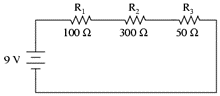Physics
Easy

Question

# Calculate the equivalent resistance of the circuit given below.## 50 Ω100 Ω300 Ω450 ΩHint:

## The correct answer is: 450 Ω

### The equivalent resistance of the circuit is 450Ω. The equivalent resistance in the resistors connected in series can be calculated by the formula Req= R1+R2+R3 The R1, R2, and R3 are 100Ω, 300Ω, and 50Ω respectively. So the R= 100+ 300+ 50= 450Ω#### With Turito Foundation.#### Get an Expert Advice From Turito.